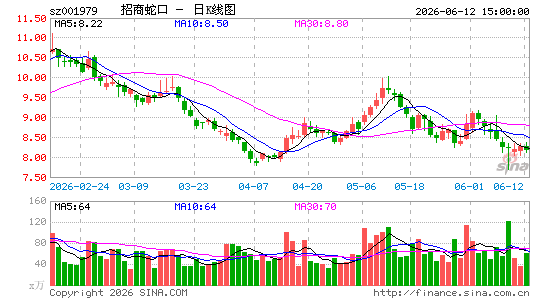•招商蛇口

,A1:=MA(C,60)>REF(MA(C,60),1);
A2:=MA(C,120)>REF(MA(C,120),1);
A3:=MA(C,240)>REF(MA(C,240),1);
A4:=A1 AND A2 AND A3 AND MA(C,60)>MA(C,120) AND MA(C,120)>MA(C,240);
XG:A4>0 AND REF(A4,1)=0;唐昭宗

﻿